# RS Aggarwal Solutions for Class 8 Maths Chapter 5 - Playing with Numbers

RS Aggarwal Solutions for Class 8 Maths Chapter 5- Playing with Numbers, are provided here. Our expert faculty team has prepared solutions in order to help you with your exam preparation to acquire good marks in Maths. Our solution module utilizes various shortcut tips and practical examples to explain all the exercise questions in a simple and easily understandable language. If you wish to secure an excellent score, solving RS Aggarwal Class 8 Solutions is an utmost necessity. These solutions will help you in gaining knowledge and strong command over the subject. Practising the textbook questions will help you in analyzing your level of preparation and knowledge of the concept.

In order to help you understand and solve the problems, we at BYJU’S have designed these solutions in a very lucid and clear manner that helps students solve problems in the most efficient possible ways. Here is the RS Aggarwal Solutions for Class 8 Chapter 5 where solutions are solved in detail. Download pdf of Class 8 Chapter 5 in their respective links.

## Download PDF of RS Aggarwal Solutions for Class 8 Maths Chapter 5 – Playing with Numbers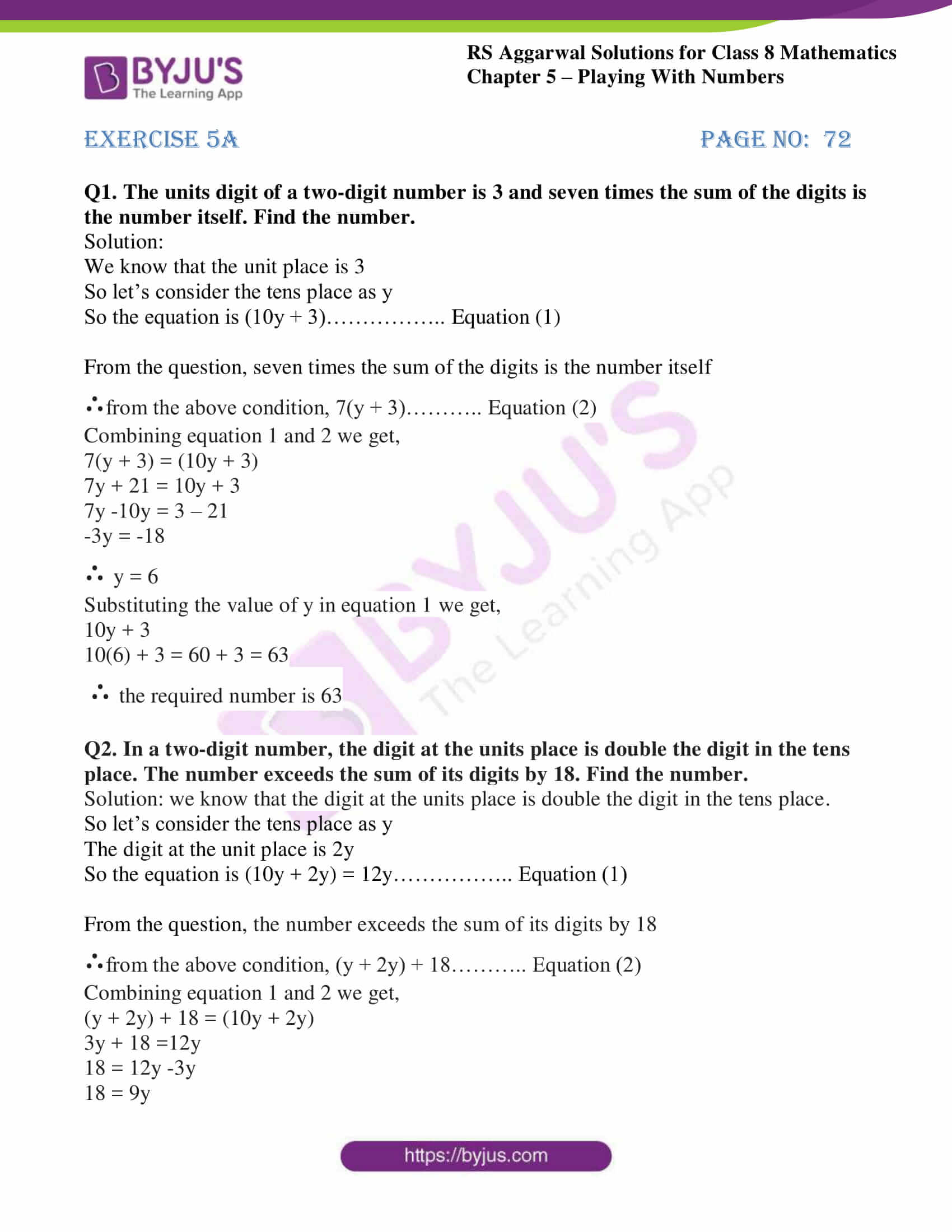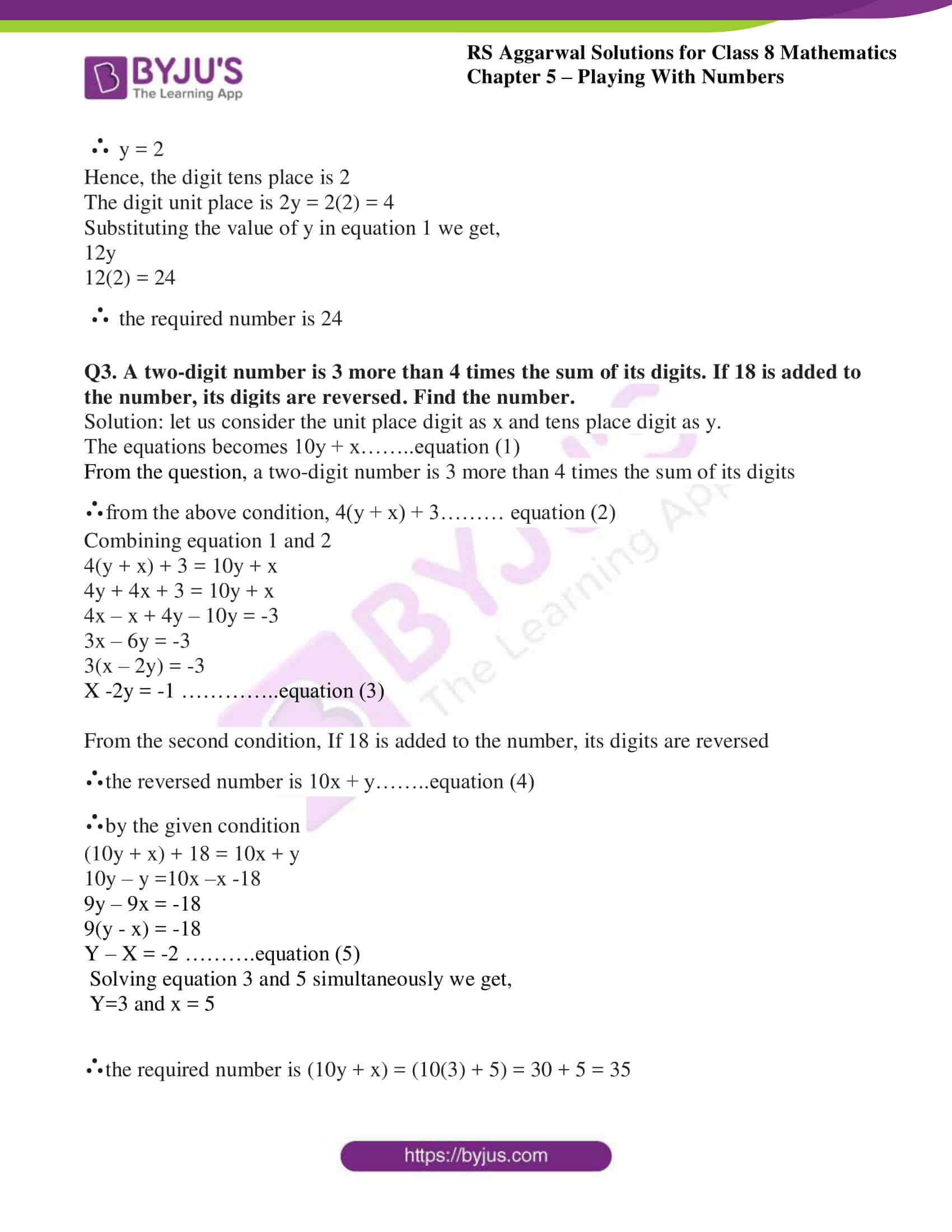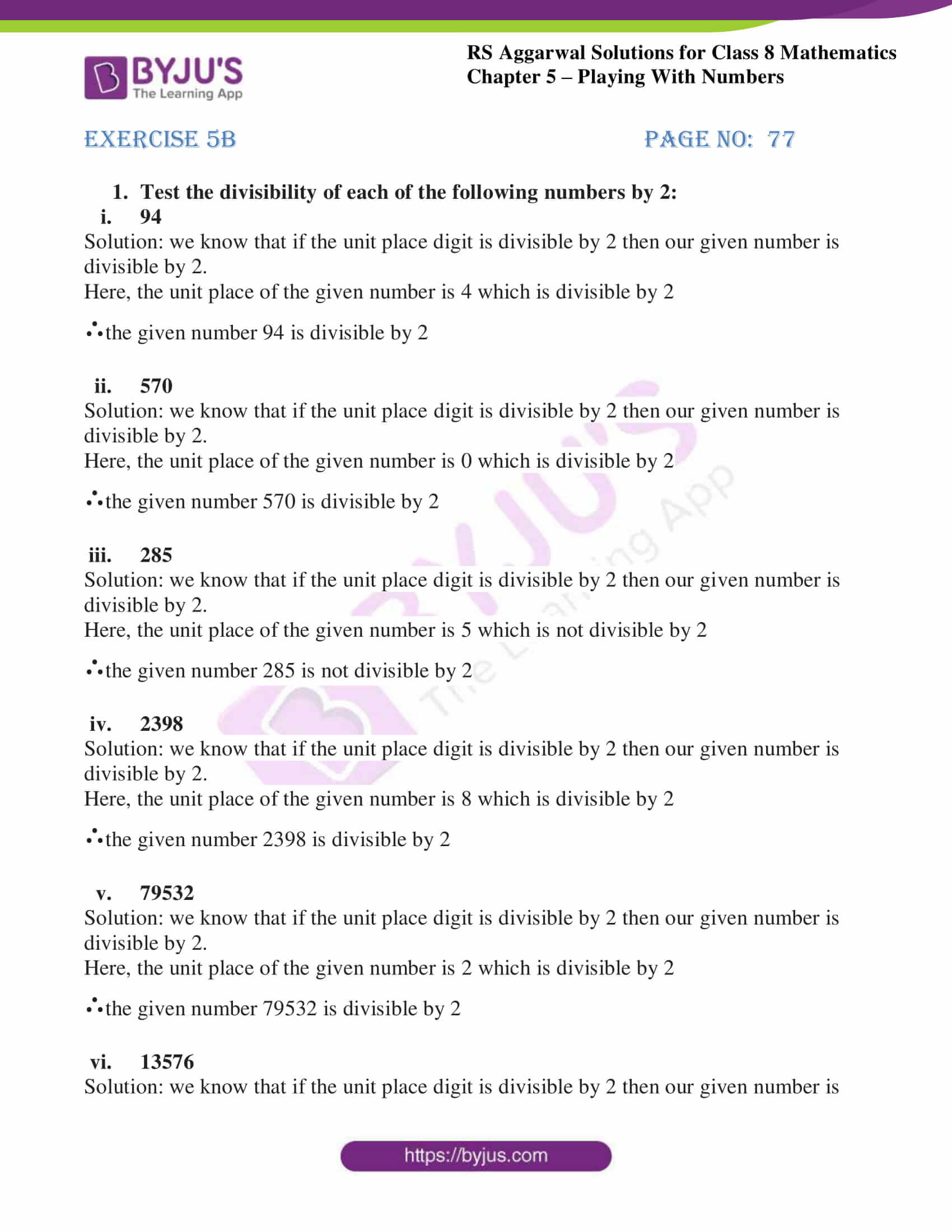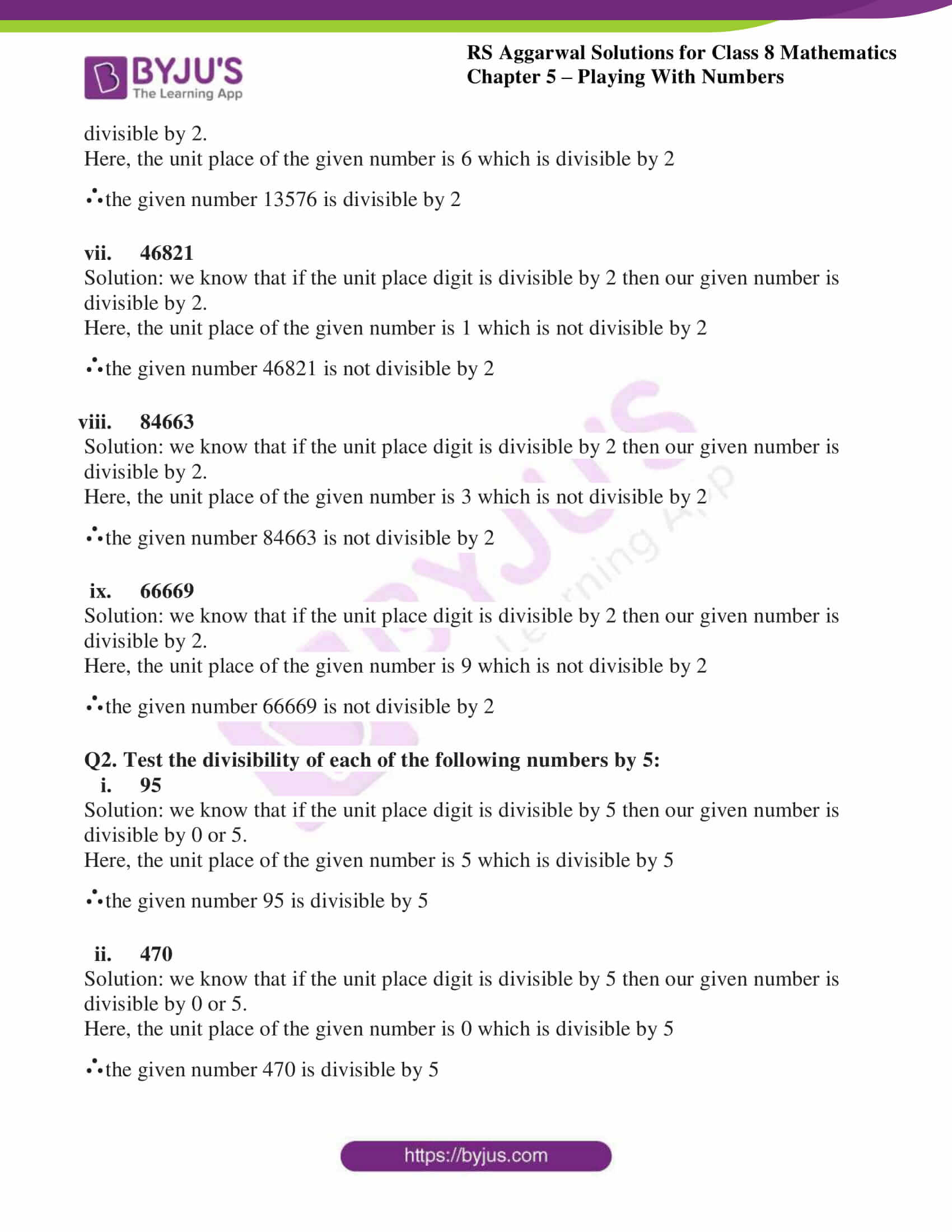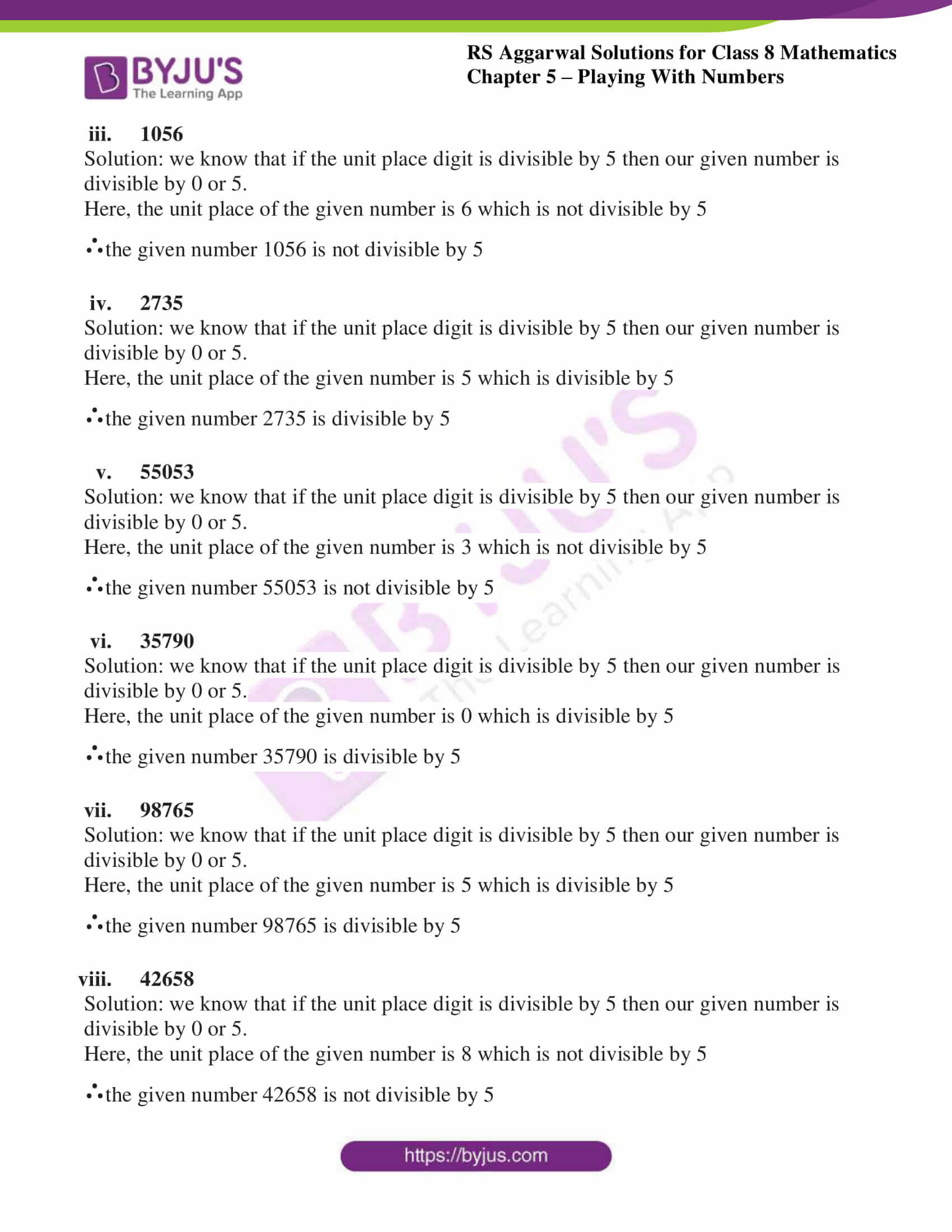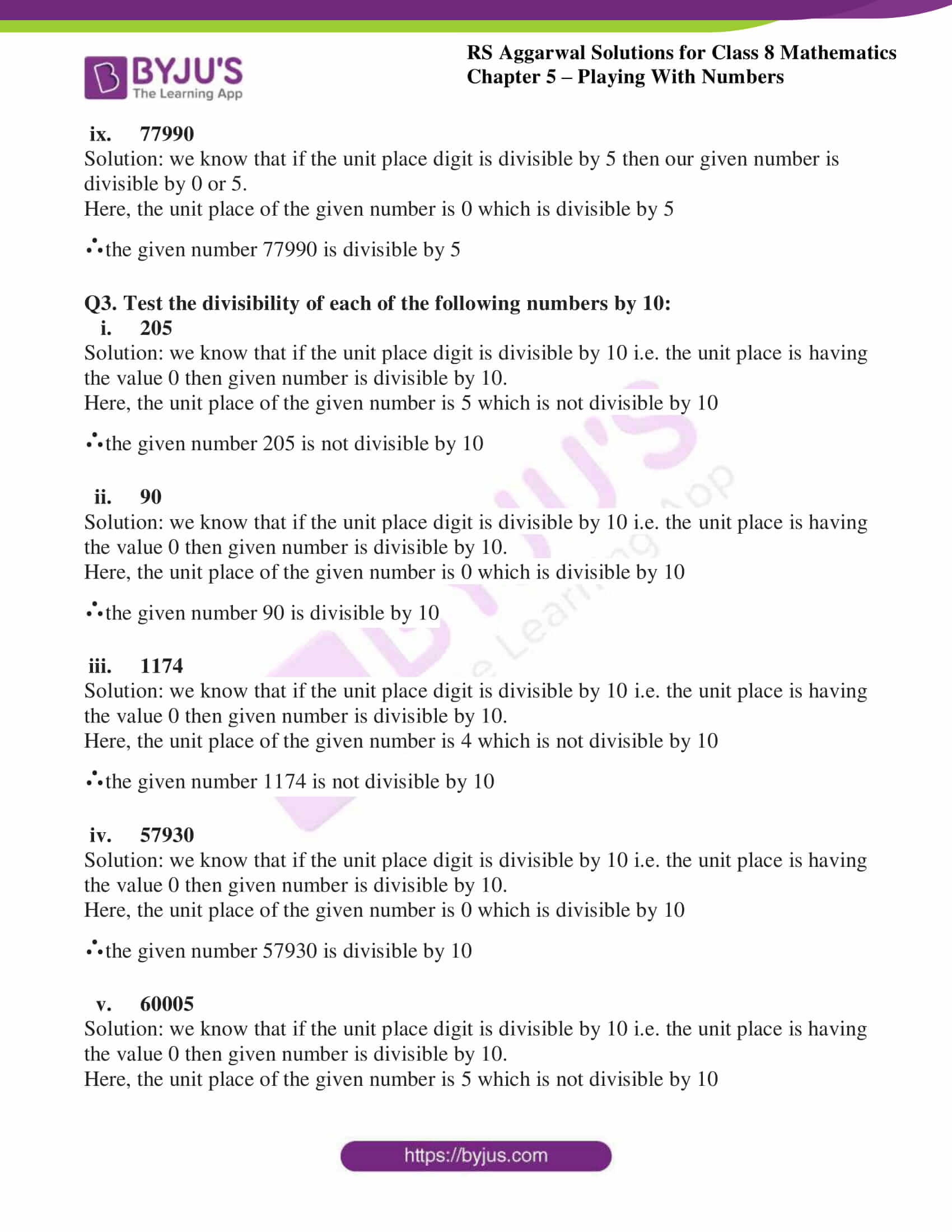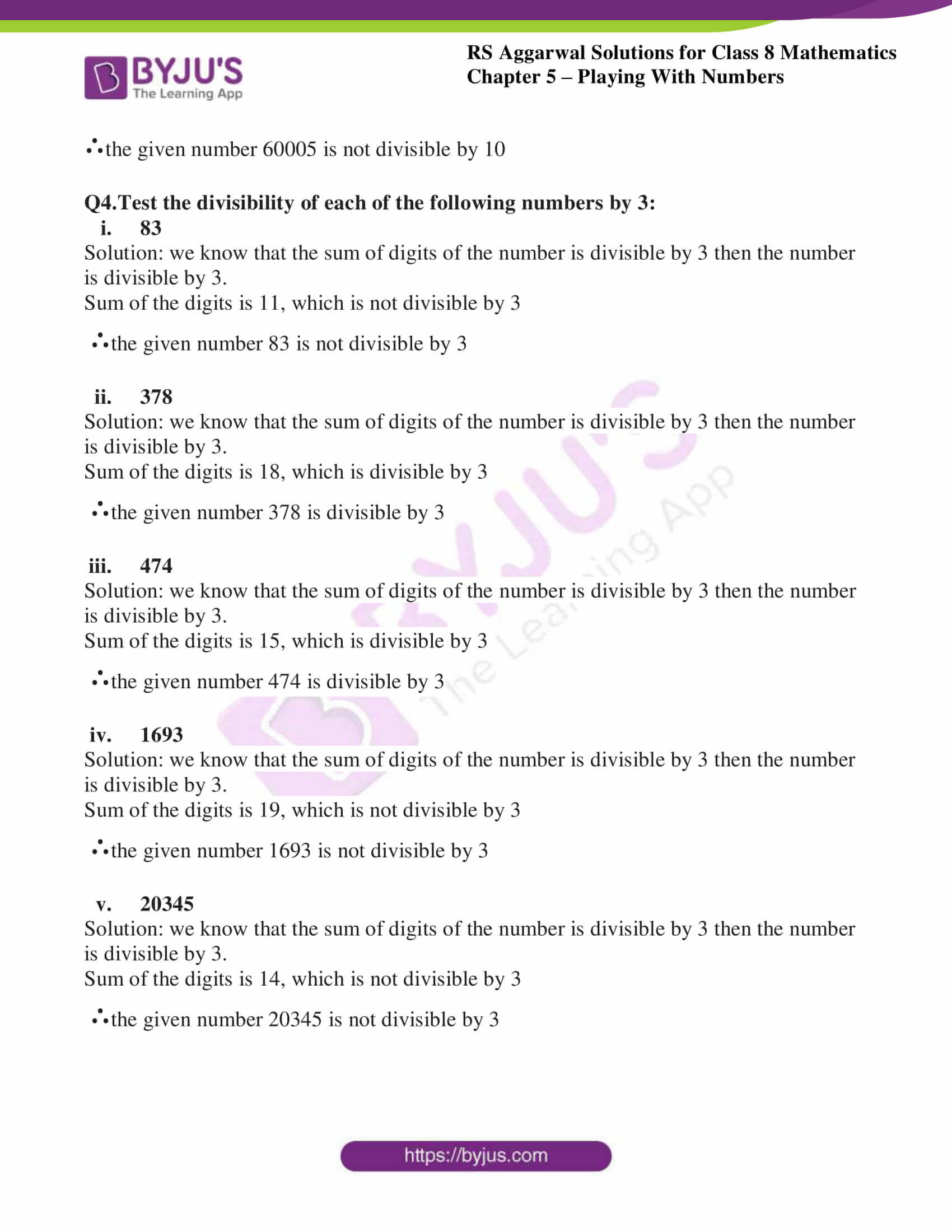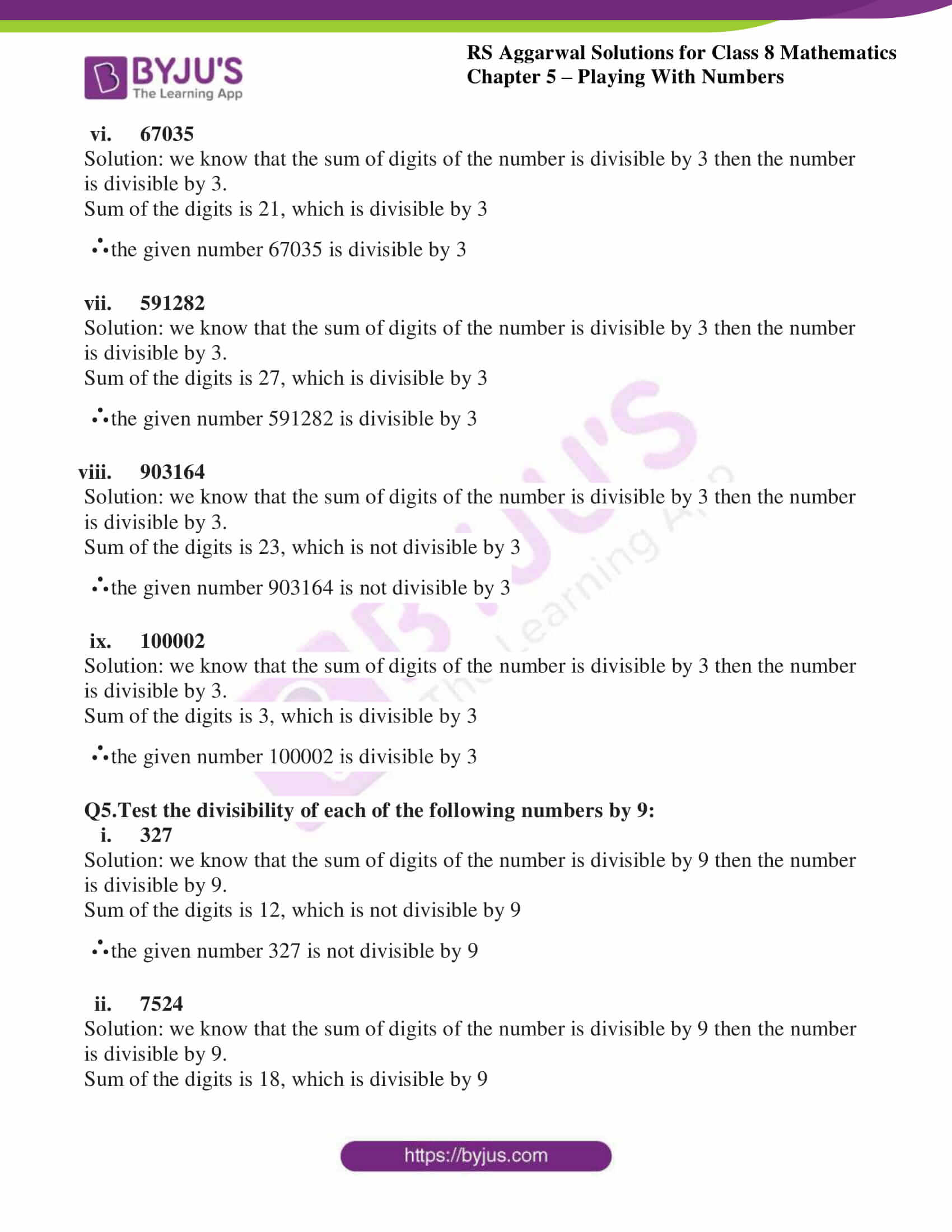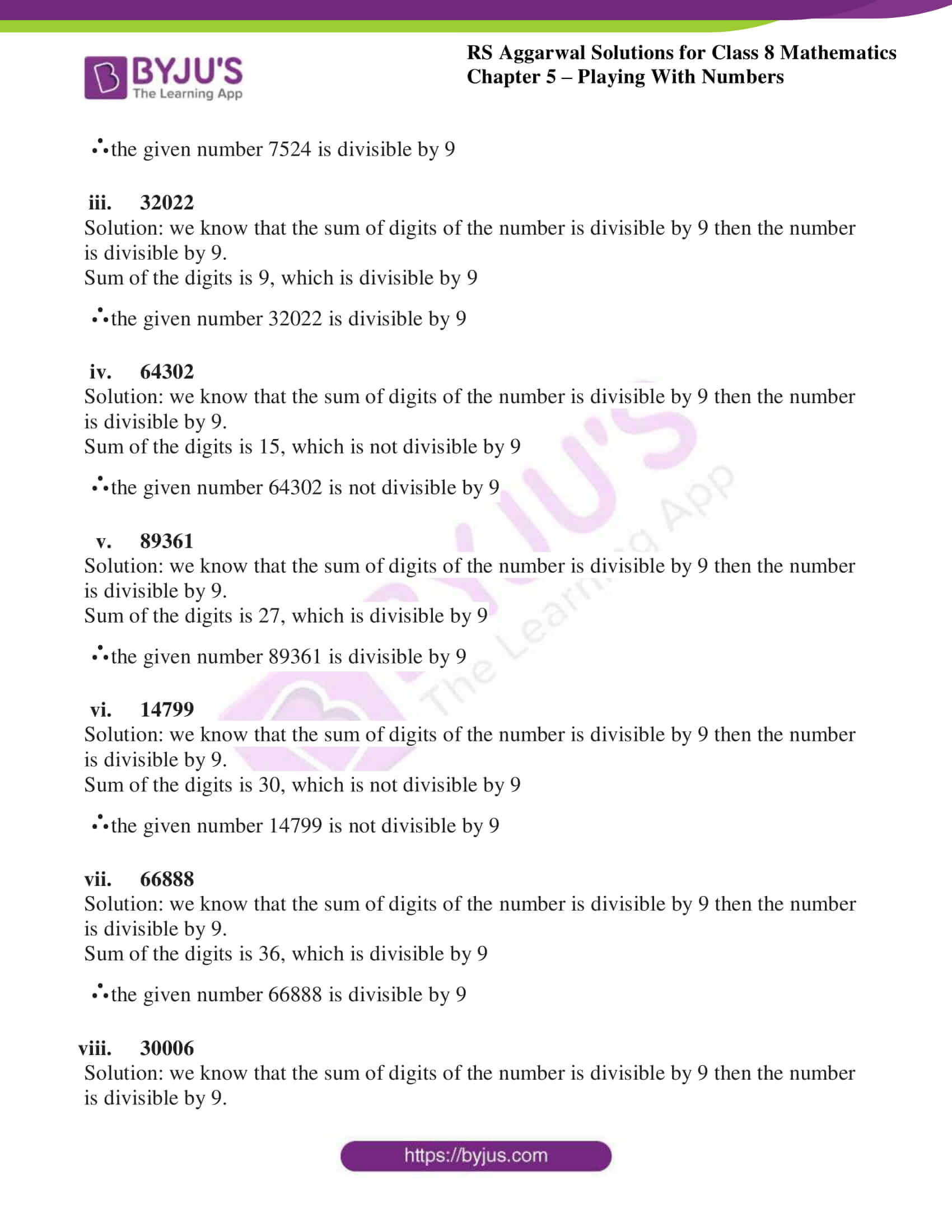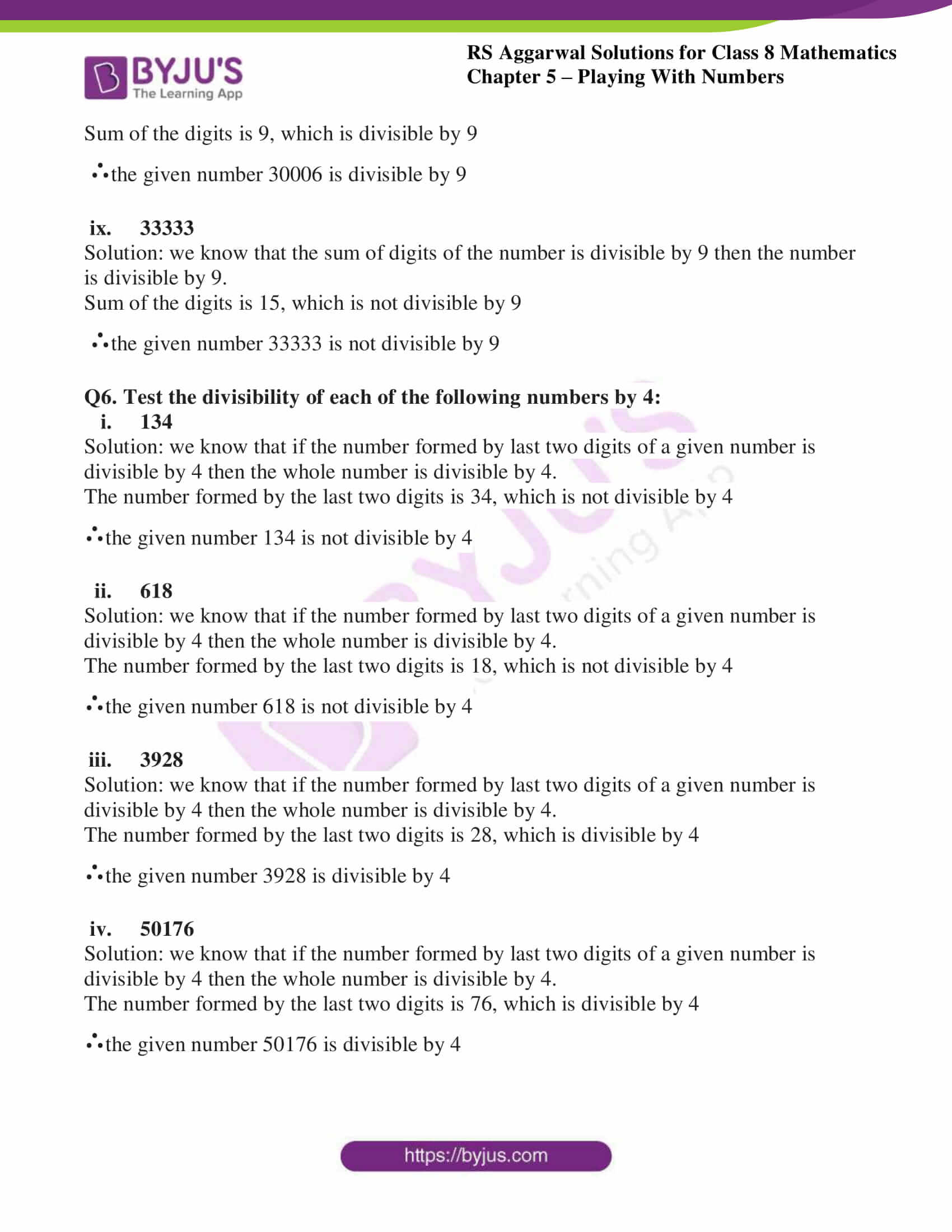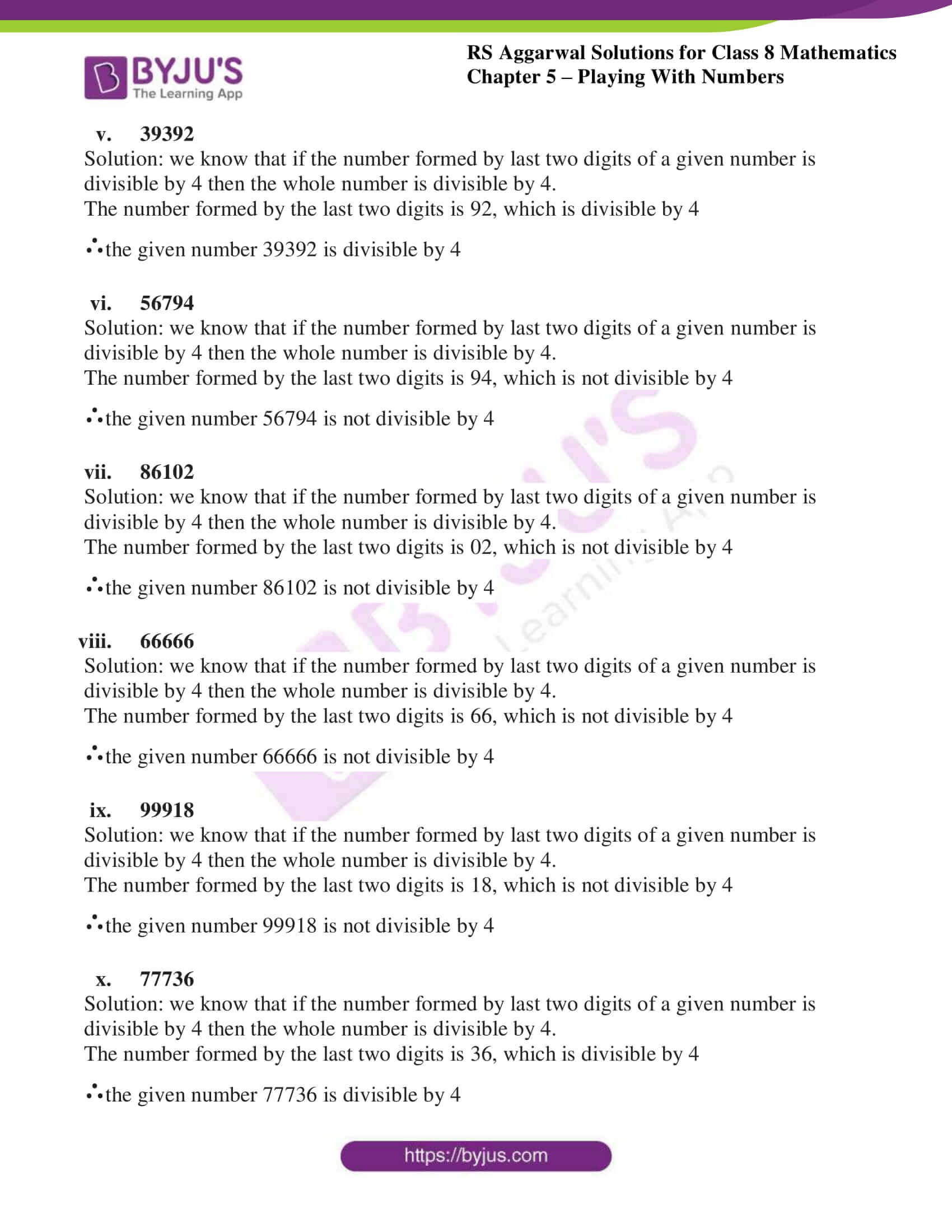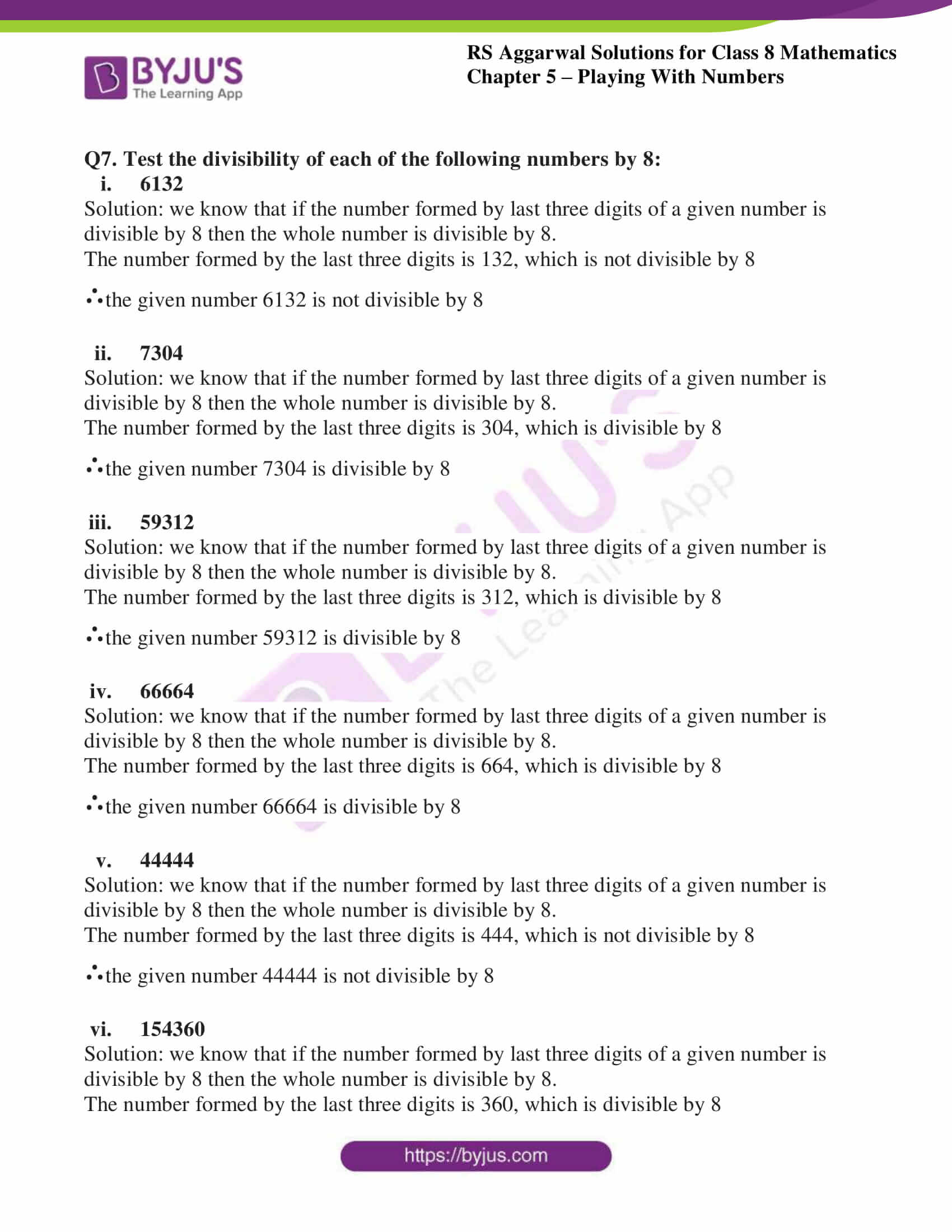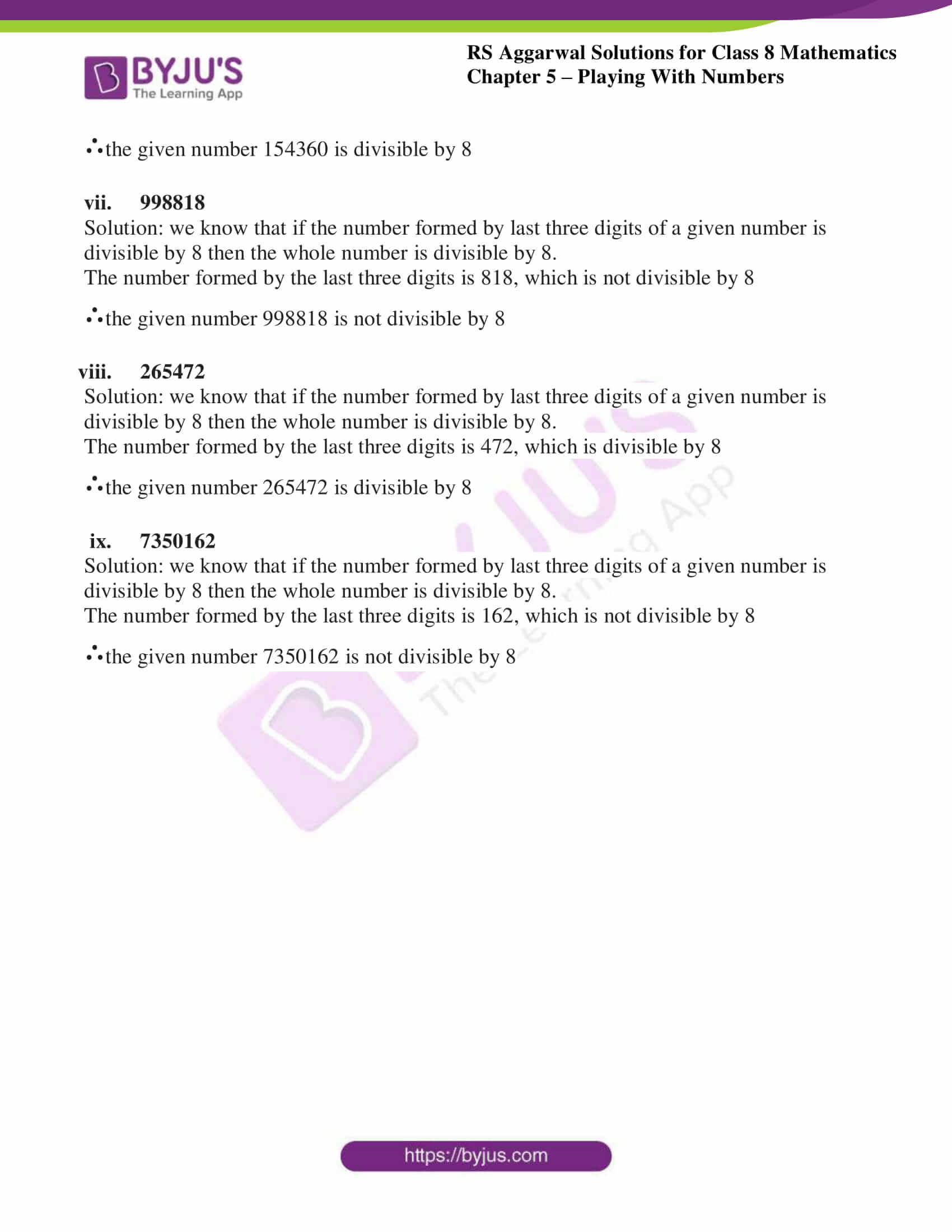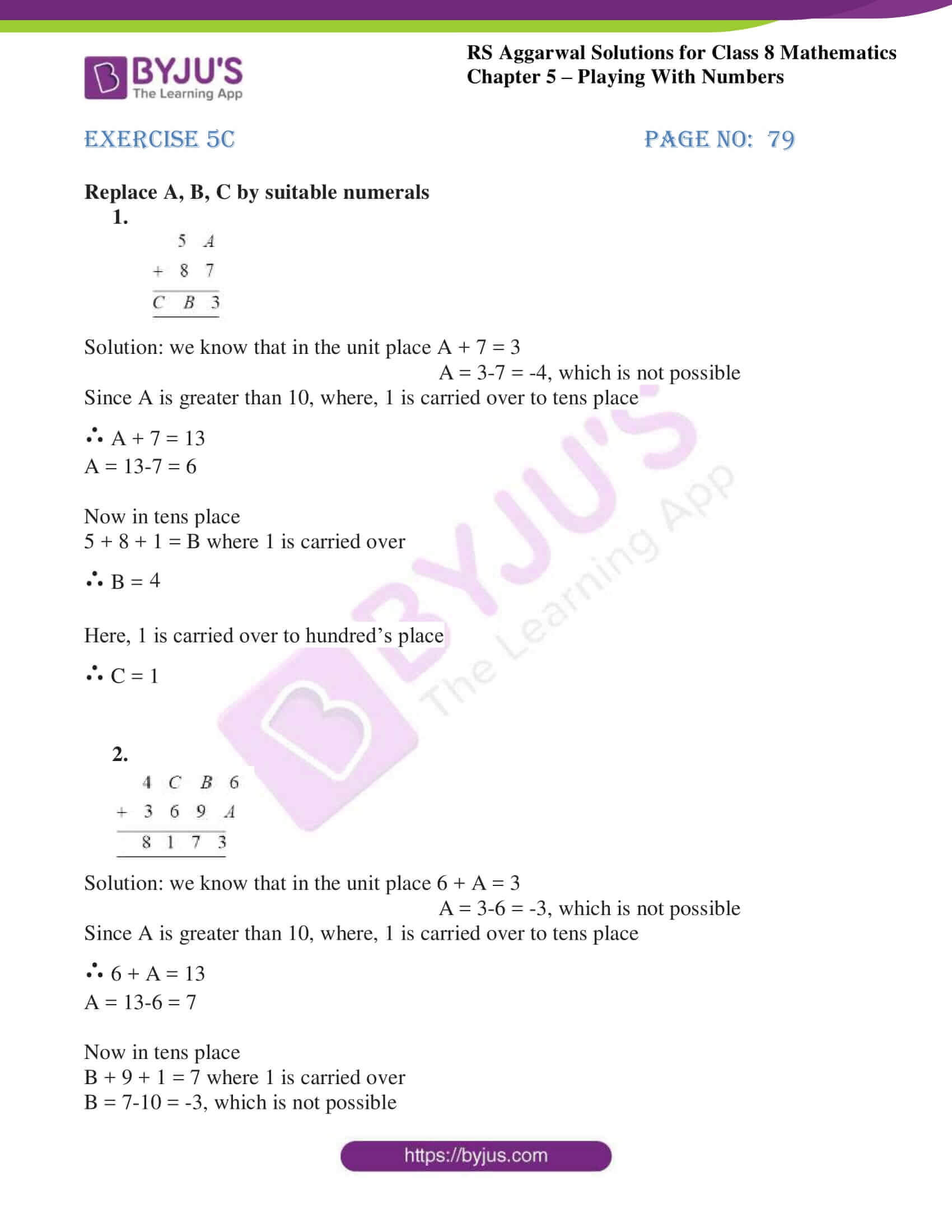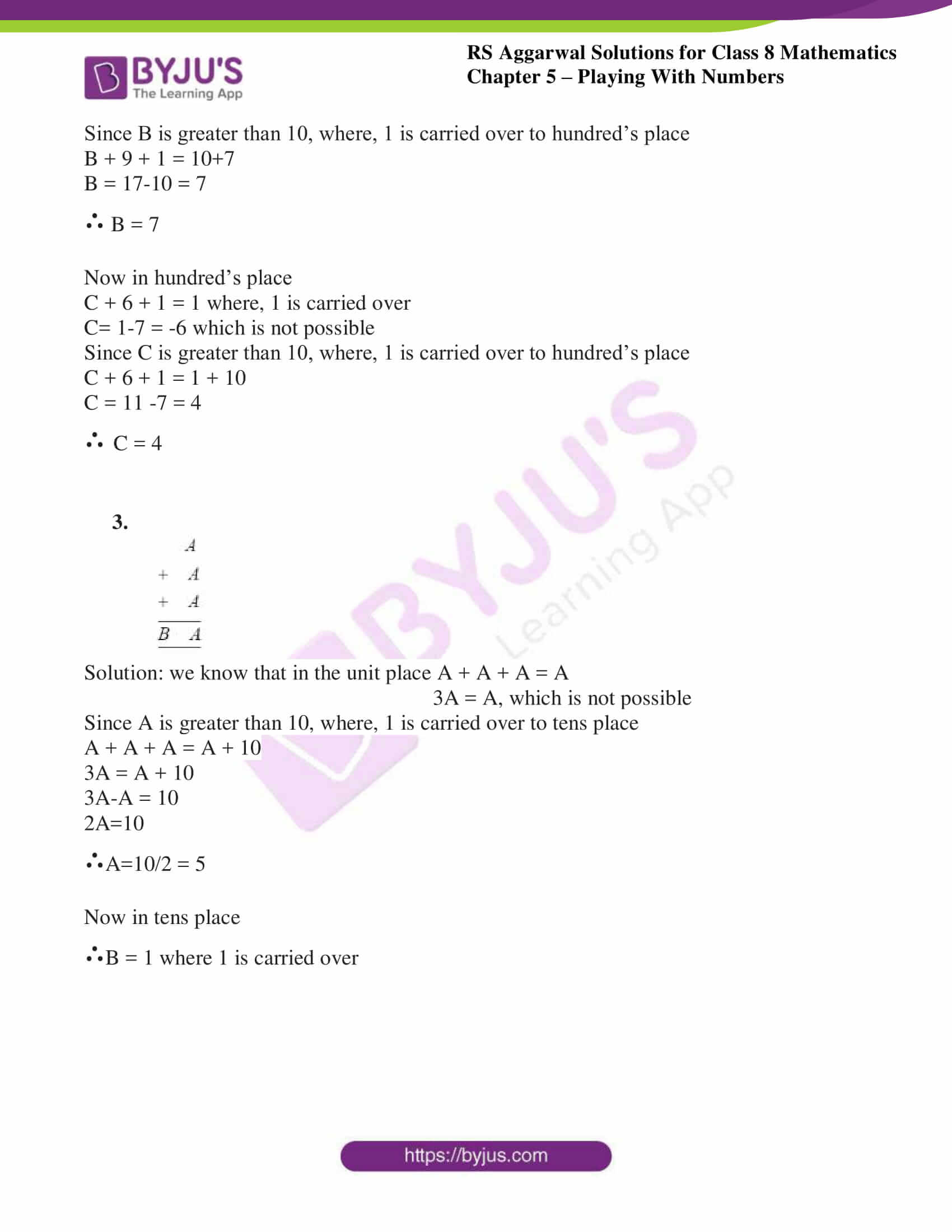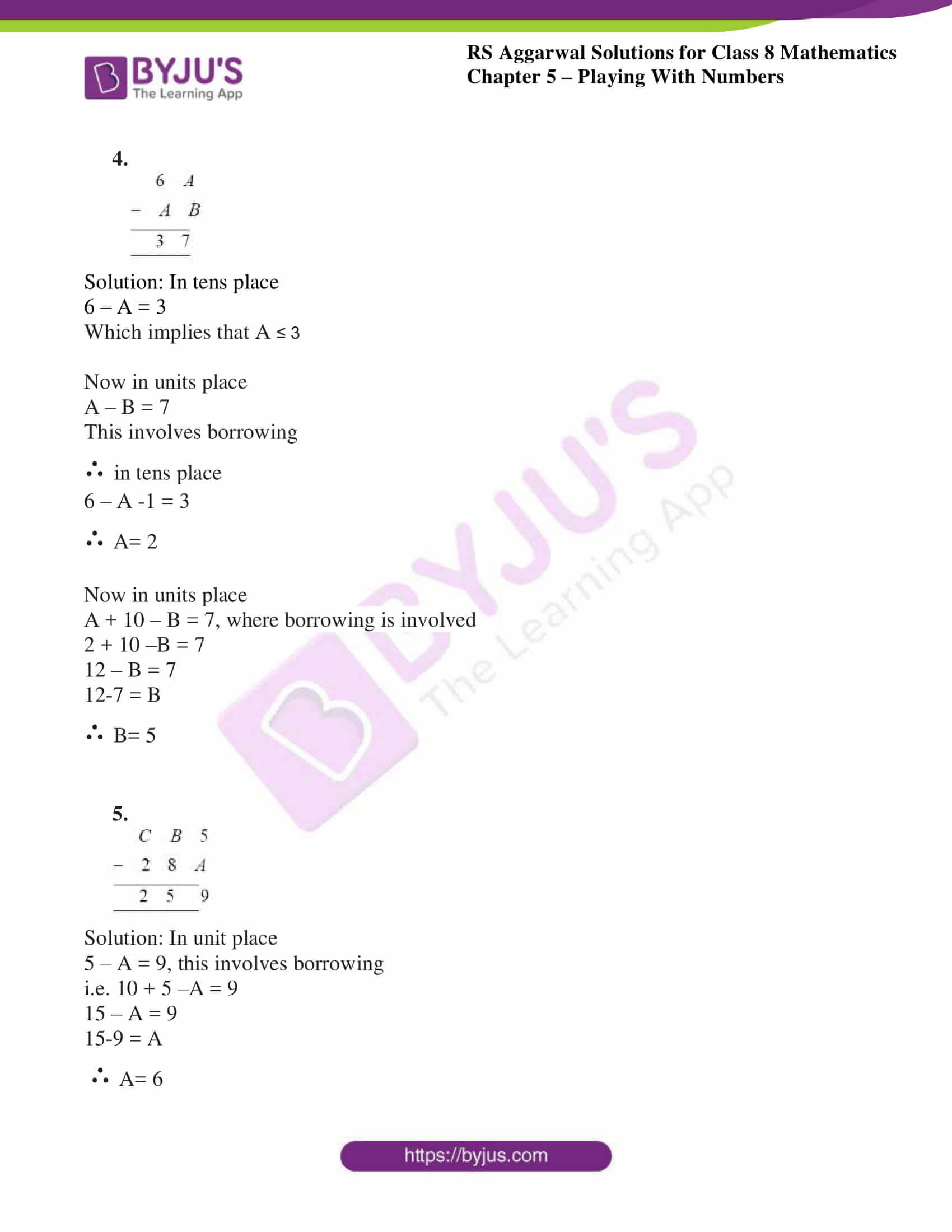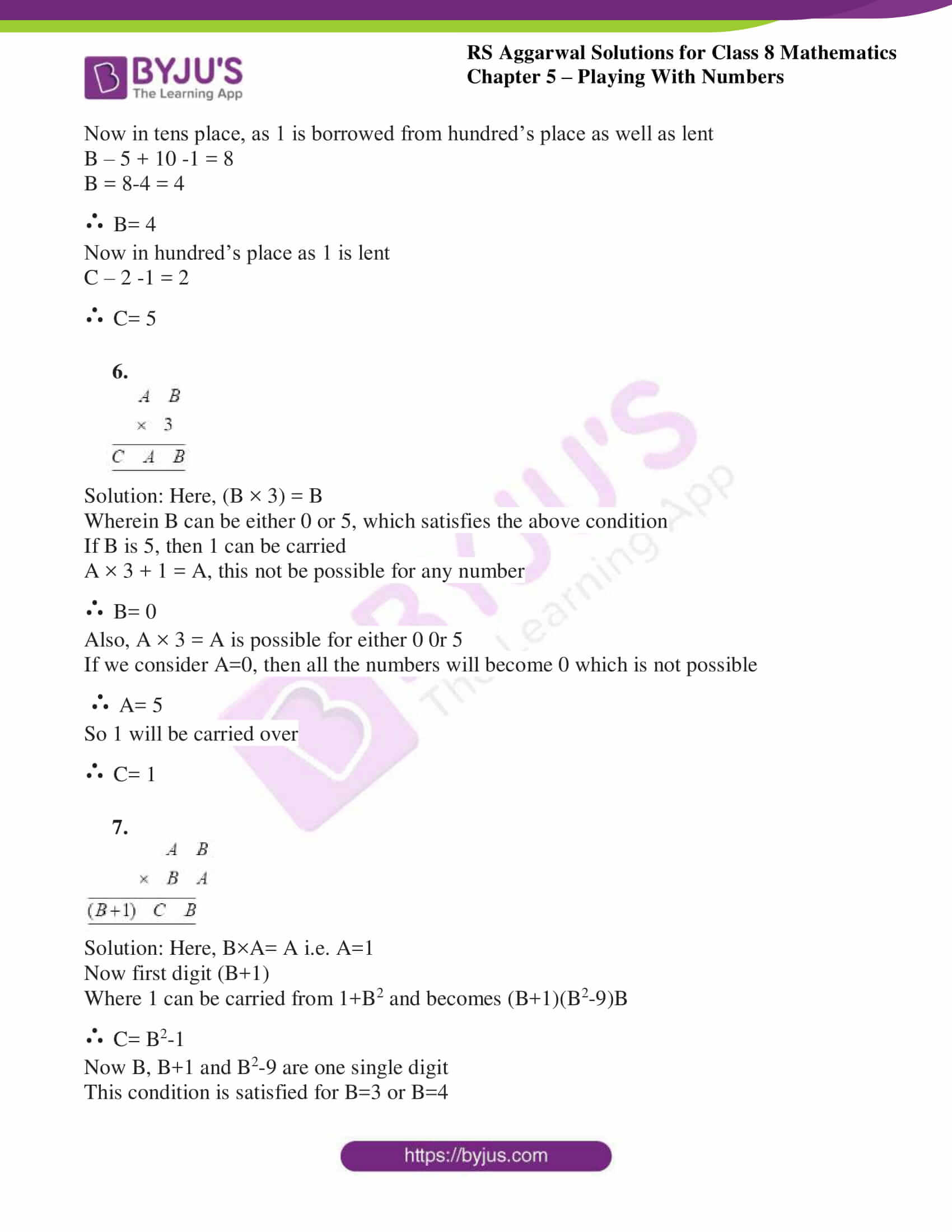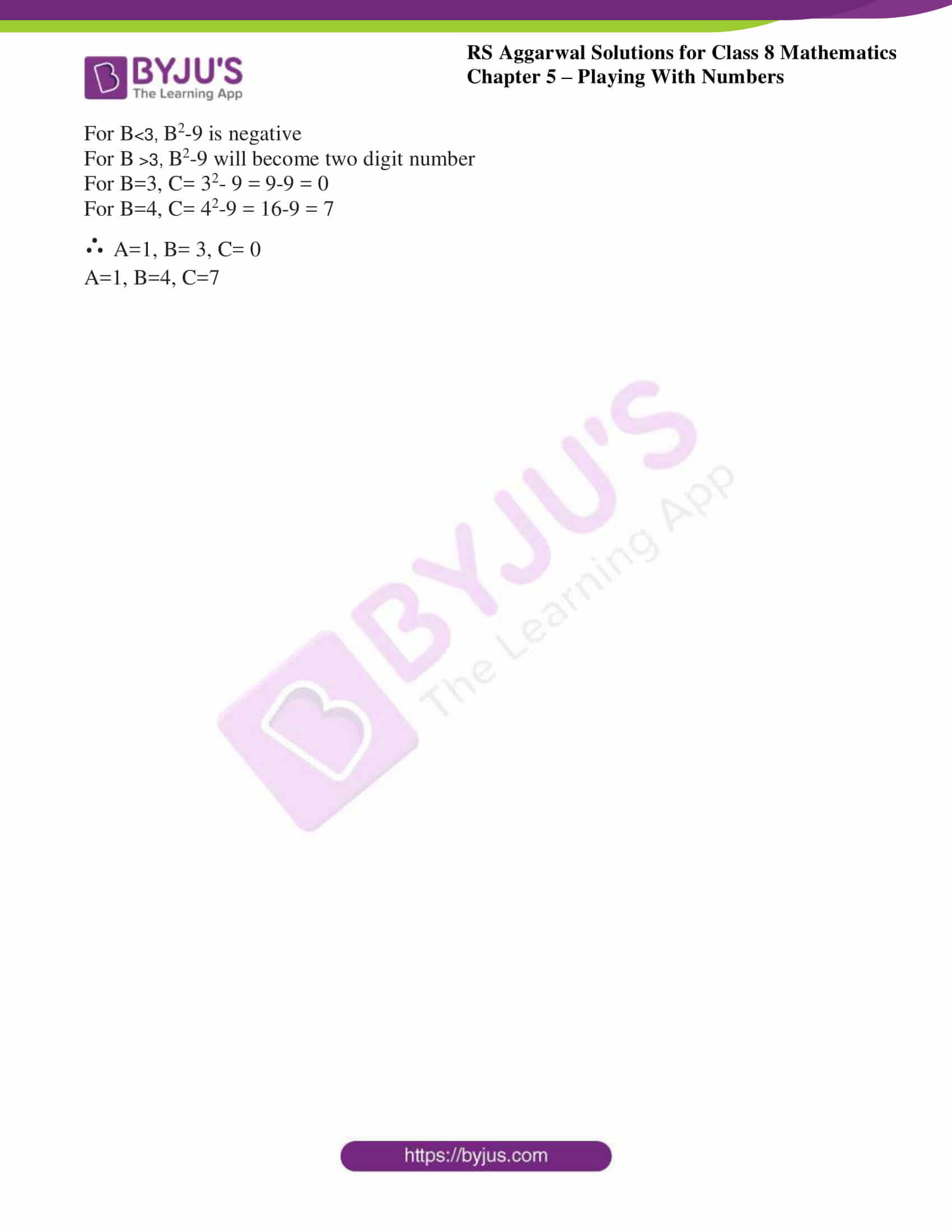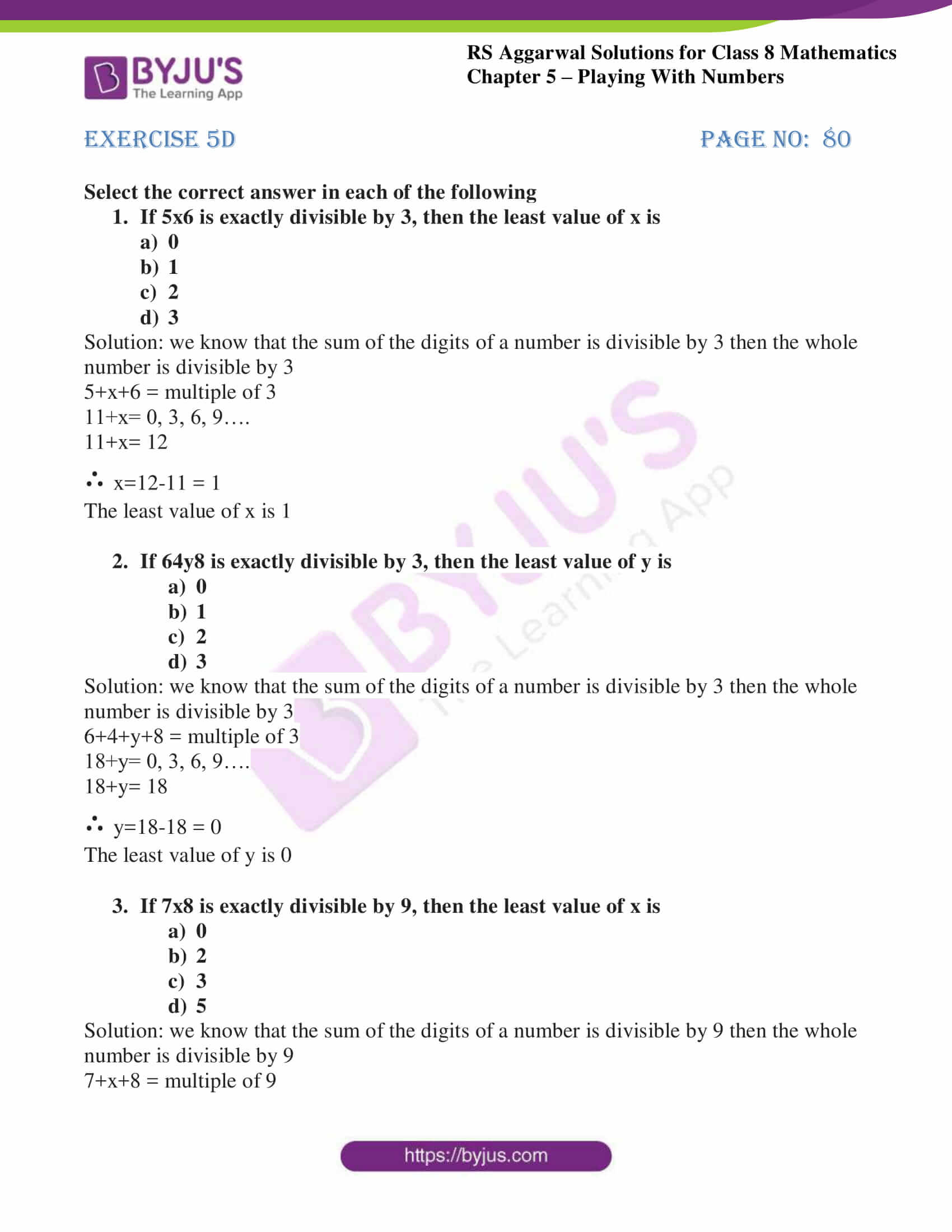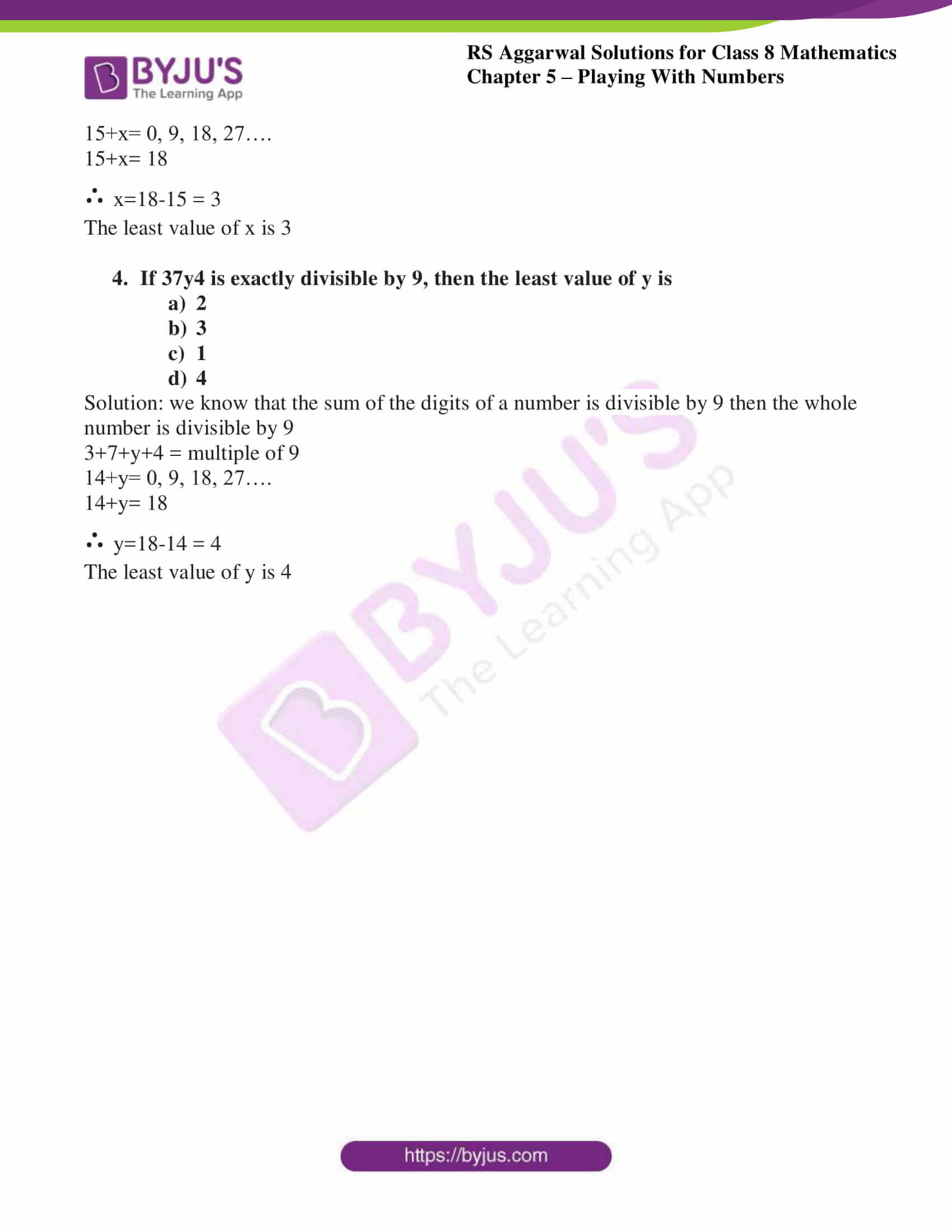## Exercise 5A

Q1. The units digit of a two-digit number is 3 and seven times the sum of the digits is the number itself. Find the number.

Solution:

We know that the unit place is 3

So let’s consider the tens place as y

So the equation is (10y + 3)…………….. Equation (1)

From the question, seven times the sum of the digits is the number itself

∴from the above condition, 7(y + 3)……….. Equation (2)

Combining equation 1 and 2 we get,

7(y + 3) = (10y + 3)

7y + 21 = 10y + 3

7y -10y = 3 – 21

-3y = -18

∴ y = 6

Substituting the value of y in equation 1 we get,

10y + 3

10(6) + 3 = 60 + 3 = 63

∴ the required number is 63

Q2. In a two-digit number, the digit at the units place is double the digit in the tens place. The number exceeds the sum of its digits by 18. Find the number.

Solution: we know that the digit at the units place is double the digit in the tens place.

So let’s consider the tens place as y

The digit at the unit place is 2y

So the equation is (10y + 2y) = 12y…………….. Equation (1)

From the question, the number exceeds the sum of its digits by 18

∴from the above condition, (y + 2y) + 18……….. Equation (2)

Combining equation 1 and 2 we get,

(y + 2y) + 18 = (10y + 2y)

3y + 18 =12y

18 = 12y -3y

18 = 9y

∴ y = 2

Hence, the digit tens place is 2

The digit unit place is 2y = 2(2) = 4

Substituting the value of y in equation 1 we get,

12y

12(2) = 24

∴ the required number is 24

Q3. A two-digit number is 3 more than 4 times the sum of its digits. If 18 is added to the number, its digits are reversed. Find the number.

Solution: let us consider the unit place digit as x and tens place digit as y.

The equations becomes 10y + x……..equation (1)

From the question, a two-digit number is 3 more than 4 times the sum of its digits

∴from the above condition, 4(y + x) + 3……… equation (2)

Combining equation 1 and 2

4(y + x) + 3 = 10y + x

4y + 4x + 3 = 10y + x

4x – x + 4y – 10y = -3

3x – 6y = -3

3(x – 2y) = -3

x -2y = -1 …………..equation (3)

From the second condition, If 18 is added to the number, its digits are reversed

∴the reversed number is 10x + y……..equation (4)

∴by the given condition

(10y + x) + 18 = 10x + y

10y – y =10x –x -18

9y – 9x = -18

9(y – x) = -18

y – x = -2 ……….equation (5)

Solving equation 3 and 5 simultaneously we get,

y=3 and x = 5

∴the required number is (10y + x) = (10(3) + 5) = 30 + 5 = 35

## Exercise 5B

Q1. Test the divisibility of each of the following numbers by 2:

i. 94

Solution: we know that if the unit place digit is divisible by 2 then our given number is divisible by 2.

Here, the unit place of the given number is 4 which is divisible by 2

∴the given number 94 is divisible by 2

ii. 570

Solution: we know that if the unit place digit is divisible by 2 then our given number is divisible by 2.

Here, the unit place of the given number is 0 which is divisible by 2

∴the given number 570 is divisible by 2

iii. 285

Solution: we know that if the unit place digit is divisible by 2 then our given number is divisible by 2.

Here, the unit place of the given number is 5 which is not divisible by 2

∴the given number 285 is not divisible by 2

iv. 2398

Solution: we know that if the unit place digit is divisible by 2 then our given number is divisible by 2.

Here, the unit place of the given number is 8 which is divisible by 2

∴the given number 2398 is divisible by 2

v. 79532

Solution: we know that if the unit place digit is divisible by 2 then our given number is divisible by 2.

Here, the unit place of the given number is 2 which is divisible by 2

∴the given number 79532 is divisible by 2

vi. 13576

Solution: we know that if the unit place digit is divisible by 2 then our given number is divisible by 2.

Here, the unit place of the given number is 6 which is divisible by 2

∴the given number 13576 is divisible by 2

vii. 46821

Solution: we know that if the unit place digit is divisible by 2 then our given number is divisible by 2.

Here, the unit place of the given number is 1 which is not divisible by 2

∴the given number 46821 is not divisible by 2

viii. 84663

Solution: we know that if the unit place digit is divisible by 2 then our given number is divisible by 2.

Here, the unit place of the given number is 3 which is not divisible by 2

∴the given number 84663 is not divisible by 2

ix. 66669

Solution: we know that if the unit place digit is divisible by 2 then our given number is divisible by 2.

Here, the unit place of the given number is 9 which is not divisible by 2

∴the given number 66669 is not divisible by 2

Q2. Test the divisibility of each of the following numbers by 5:

i. 95

Solution: we know that if the unit place digit is divisible by 5 then our given number is divisible by 0 or 5.

Here, the unit place of the given number is 5 which is divisible by 5

∴the given number 95 is divisible by 5

ii. 470

Solution: we know that if the unit place digit is divisible by 5 then our given number is divisible by 0 or 5.

Here, the unit place of the given number is 0 which is divisible by 5

∴the given number 470 is divisible by 5

iii. 1056

Solution: we know that if the unit place digit is divisible by 5 then our given number is divisible by 0 or 5.

Here, the unit place of the given number is 6 which is not divisible by 5

∴the given number 1056 is not divisible by 5

iv. 2735

Solution: we know that if the unit place digit is divisible by 5 then our given number is divisible by 0 or 5.

Here, the unit place of the given number is 5 which is divisible by 5

∴the given number 2735 is divisible by 5

v. 55053

Solution: we know that if the unit place digit is divisible by 5 then our given number is divisible by 0 or 5.

Here, the unit place of the given number is 3 which is not divisible by 5

∴the given number 55053 is not divisible by 5

vi. 35790

Solution: we know that if the unit place digit is divisible by 5 then our given number is divisible by 0 or 5.

Here, the unit place of the given number is 0 which is divisible by 5

∴the given number 35790 is divisible by 5

vii. 98765

Solution: we know that if the unit place digit is divisible by 5 then our given number is divisible by 0 or 5.

Here, the unit place of the given number is 5 which is divisible by 5

∴the given number 98765 is divisible by 5

viii. 42658

Solution: we know that if the unit place digit is divisible by 5 then our given number is divisible by 0 or 5.

Here, the unit place of the given number is 8 which is not divisible by 5

∴the given number 42658 is not divisible by 5

ix. 77990

Solution: we know that if the unit place digit is divisible by 5 then our given number is divisible by 0 or 5.

Here, the unit place of the given number is 0 which is divisible by 5

∴the given number 77990 is divisible by 5

Q3. Test the divisibility of each of the following numbers by 10:

i. 205

Solution: we know that if the unit place digit is divisible by 10 i.e. the unit place is having the value 0 then given number is divisible by 10.

Here, the unit place of the given number is 5 which is not divisible by 10

∴the given number 205 is not divisible by 10

ii. 90

Solution: we know that if the unit place digit is divisible by 10 i.e. the unit place is having the value 0 then given number is divisible by 10.

Here, the unit place of the given number is 0 which is divisible by 10

∴the given number 90 is divisible by 10

iii. 1174

Solution: we know that if the unit place digit is divisible by 10 i.e. the unit place is having the value 0 then given number is divisible by 10.

Here, the unit place of the given number is 4 which is not divisible by 10

∴the given number 1174 is not divisible by 10

iv. 57930

Solution: we know that if the unit place digit is divisible by 10 i.e. the unit place is having the value 0 then given number is divisible by 10.

Here, the unit place of the given number is 0 which is divisible by 10

∴the given number 57930 is divisible by 10

v. 60005

Solution: we know that if the unit place digit is divisible by 10 i.e. the unit place is having the value 0 then given number is divisible by 10.

Here, the unit place of the given number is 5 which is not divisible by 10

∴the given number 60005 is not divisible by 10

Q4.Test the divisibility of each of the following numbers by 3:

i. 83

Solution: we know that the sum of digits of the number is divisible by 3 then the number is divisible by 3.

Sum of the digits is 11, which is not divisible by 3

∴the given number 83 is not divisible by 3

ii. 378

Solution: we know that the sum of digits of the number is divisible by 3 then the number is divisible by 3.

Sum of the digits is 18, which is divisible by 3

∴the given number 378 is divisible by 3

iii. 474

Solution: we know that the sum of digits of the number is divisible by 3 then the number is divisible by 3.

Sum of the digits is 15, which is divisible by 3

∴the given number 474 is divisible by 3

iv. 1693

Solution: we know that the sum of digits of the number is divisible by 3 then the number is divisible by 3.

Sum of the digits is 19, which is not divisible by 3

∴the given number 1693 is not divisible by 3

v. 20345

Solution: we know that the sum of digits of the number is divisible by 3 then the number is divisible by 3.

Sum of the digits is 14, which is not divisible by 3

∴the given number 20345 is not divisible by 3

vi. 67035

Solution: we know that the sum of digits of the number is divisible by 3 then the number is divisible by 3.

Sum of the digits is 21, which is divisible by 3

∴the given number 67035 is divisible by 3

vii. 591282

Solution: we know that the sum of digits of the number is divisible by 3 then the number is divisible by 3.

Sum of the digits is 27, which is divisible by 3

∴the given number 591282 is divisible by 3

viii. 903164

Solution: we know that the sum of digits of the number is divisible by 3 then the number is divisible by 3.

Sum of the digits is 23, which is not divisible by 3

∴the given number 903164 is not divisible by 3

ix. 100002

Solution: we know that the sum of digits of the number is divisible by 3 then the number is divisible by 3.

Sum of the digits is 3, which is divisible by 3

∴the given number 100002 is divisible by 3

Q5.Test the divisibility of each of the following numbers by 9:

i. 327

Solution: we know that the sum of digits of the number is divisible by 9 then the number is divisible by 9.

Sum of the digits is 12, which is not divisible by 9

∴the given number 327 is not divisible by 9

ii. 7524

Solution: we know that the sum of digits of the number is divisible by 9 then the number is divisible by 9.

Sum of the digits is 18, which is divisible by 9

∴the given number 7524 is divisible by 9

iii. 32022

Solution: we know that the sum of digits of the number is divisible by 9 then the number is divisible by 9.

Sum of the digits is 9, which is divisible by 9

∴the given number 32022 is divisible by 9

iv. 64302

Solution: we know that the sum of digits of the number is divisible by 9 then the number is divisible by 9.

Sum of the digits is 15, which is not divisible by 9

∴the given number 64302 is not divisible by 9

v. 89361

Solution: we know that the sum of digits of the number is divisible by 9 then the number is divisible by 9.

Sum of the digits is 27, which is divisible by 9

∴the given number 89361 is divisible by 9

vi. 14799

Solution: we know that the sum of digits of the number is divisible by 9 then the number is divisible by 9.

Sum of the digits is 30, which is not divisible by 9

∴the given number 14799 is not divisible by 9

vii. 66888

Solution: we know that the sum of digits of the number is divisible by 9 then the number is divisible by 9.

Sum of the digits is 36, which is divisible by 9

∴the given number 66888 is divisible by 9

viii. 30006

Solution: we know that the sum of digits of the number is divisible by 9 then the number is divisible by 9.

Sum of the digits is 9, which is divisible by 9

∴the given number 30006 is divisible by 9

ix. 33333

Solution: we know that the sum of digits of the number is divisible by 9 then the number is divisible by 9.

Sum of the digits is 15, which is not divisible by 9

∴the given number 33333 is not divisible by 9

Q6. Test the divisibility of each of the following numbers by 4:

i. 134

Solution: we know that if the number formed by last two digits of a given number is divisible by 4 then the whole number is divisible by 4.

The number formed by the last two digits is 34, which is not divisible by 4

∴the given number 134 is not divisible by 4

ii. 618

Solution: we know that if the number formed by last two digits of a given number is divisible by 4 then the whole number is divisible by 4.

The number formed by the last two digits is 18, which is not divisible by 4

∴the given number 618 is not divisible by 4

iii. 3928

Solution: we know that if the number formed by last two digits of a given number is divisible by 4 then the whole number is divisible by 4.

The number formed by the last two digits is 28, which is divisible by 4

∴the given number 3928 is divisible by 4

iv. 50176

Solution: we know that if the number formed by last two digits of a given number is divisible by 4 then the whole number is divisible by 4.

The number formed by the last two digits is 76, which is divisible by 4

∴the given number 50176 is divisible by 4

v. 39392

Solution: we know that if the number formed by last two digits of a given number is divisible by 4 then the whole number is divisible by 4.

The number formed by the last two digits is 92, which is divisible by 4

∴the given number 39392 is divisible by 4

vi. 56794

Solution: we know that if the number formed by last two digits of a given number is divisible by 4 then the whole number is divisible by 4.

The number formed by the last two digits is 94, which is not divisible by 4

∴the given number 56794 is not divisible by 4

vii. 86102

Solution: we know that if the number formed by last two digits of a given number is divisible by 4 then the whole number is divisible by 4.

The number formed by the last two digits is 02, which is not divisible by 4

∴the given number 86102 is not divisible by 4

viii. 66666

Solution: we know that if the number formed by last two digits of a given number is divisible by 4 then the whole number is divisible by 4.

The number formed by the last two digits is 66, which is not divisible by 4

∴the given number 66666 is not divisible by 4

ix. 99918

Solution: we know that if the number formed by last two digits of a given number is divisible by 4 then the whole number is divisible by 4.

The number formed by the last two digits is 18, which is not divisible by 4

∴the given number 99918 is not divisible by 4

x. 77736

Solution: we know that if the number formed by last two digits of a given number is divisible by 4 then the whole number is divisible by 4.

The number formed by the last two digits is 36, which is divisible by 4

∴the given number 77736 is divisible by 4

Q7. Test the divisibility of each of the following numbers by 8:

i. 6132

Solution: we know that if the number formed by last three digits of a given number is divisible by 8 then the whole number is divisible by 8.

The number formed by the last three digits is 132, which is not divisible by 8

∴the given number 6132 is not divisible by 8

ii. 7304

Solution: we know that if the number formed by last three digits of a given number is divisible by 8 then the whole number is divisible by 8.

The number formed by the last three digits is 304, which is divisible by 8

∴the given number 7304 is divisible by 8

iii. 59312

Solution: we know that if the number formed by last three digits of a given number is divisible by 8 then the whole number is divisible by 8.

The number formed by the last three digits is 312, which is divisible by 8

∴the given number 59312 is divisible by 8

iv. 66664

Solution: we know that if the number formed by last three digits of a given number is divisible by 8 then the whole number is divisible by 8.

The number formed by the last three digits is 664, which is divisible by 8

∴the given number 66664 is divisible by 8

v. 44444

Solution: we know that if the number formed by last three digits of a given number is divisible by 8 then the whole number is divisible by 8.

The number formed by the last three digits is 444, which is not divisible by 8

∴the given number 44444 is not divisible by 8

vi. 154360

Solution: we know that if the number formed by last three digits of a given number is divisible by 8 then the whole number is divisible by 8.

The number formed by the last three digits is 360, which is divisible by 8

∴the given number 154360 is divisible by 8

vii. 998818

Solution: we know that if the number formed by last three digits of a given number is divisible by 8 then the whole number is divisible by 8.

The number formed by the last three digits is 818, which is not divisible by 8

∴the given number 998818 is not divisible by 8

viii. 265472

Solution: we know that if the number formed by last three digits of a given number is divisible by 8 then the whole number is divisible by 8.

The number formed by the last three digits is 472, which is divisible by 8

∴the given number 265472 is divisible by 8

ix. 7350162

Solution: we know that if the number formed by last three digits of a given number is divisible by 8 then the whole number is divisible by 8.

The number formed by the last three digits is 162, which is not divisible by 8

∴the given number 7350162 is not divisible by 8

## Exercise 5C

Replace A, B, C by suitable numerals

Q1.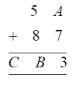Solution: we know that in the unit place A + 7 = 3

A = 3-7 = -4, which is not possible

Since A is greater than 10, where, 1 is carried over to tens place

∴ A + 7 = 13

A = 13-7 = 6

Now in tens place

5 + 8 + 1 = B where 1 is carried over

∴ B = 4

Here, 1 is carried over to hundred’s place

∴ C = 1

Q2.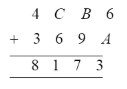Solution: we know that in the unit place 6 + A = 3

A = 3-6 = -3, which is not possible

Since A is greater than 10, where, 1 is carried over to tens place

∴ 6 + A = 13

A = 13-6 = 7

Now in tens place

B + 9 + 1 = 7 where 1 is carried over

B = 7-10 = -3, which is not possible

Since B is greater than 10, where, 1 is carried over to hundred’s place

B + 9 + 1 = 10+7

B = 17-10 = 7

∴ B = 7

Now in hundred’s place

C + 6 + 1 = 1 where, 1 is carried over

C= 1-7 = -6 which is not possible

Since C is greater than 10, where, 1 is carried over to hundred’s place

C + 6 + 1 = 1 + 10

C = 11 -7 = 4

∴ C = 4

Q3.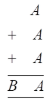Solution: we know that in the unit place A + A + A = A

3A = A, which is not possible

Since A is greater than 10, where, 1 is carried over to tens place

A + A + A = A + 10

3A = A + 10

3A-A = 10

2A=10

∴A=10/2 = 5

Now in tens place

∴B = 1 where 1 is carried over

Q4.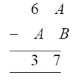Solution: In tens place

6 – A = 3

Which implies that A ≤ 3

Now in units place

A – B = 7

This involves borrowing

∴ in tens place

6 – A -1 = 3

∴ A= 2

Now in units place

A + 10 – B = 7, where borrowing is involved

2 + 10 –B = 7

12 – B = 7

12-7 = B

∴ B= 5

Q5.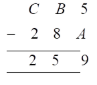Solution: In unit place

5 – A = 9, this involves borrowing

i.e. 10 + 5 –A = 9

15 – A = 9

15-9 = A

∴ A= 6

Now in tens place, as 1 is borrowed from hundred’s place as well as lent

B – 5 + 10 -1 = 8

B = 8-4 = 4

∴ B= 4

Now in hundred’s place as 1 is lent

C – 2 -1 = 2

∴ C= 5

Q6.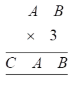Solution: Here, (B × 3) = B

Wherein B can be either 0 or 5, which satisfies the above condition

If B is 5, then 1 can be carried

A × 3 + 1 = A, this not be possible for any number

∴ B= 0

Also, A × 3 = A is possible for either 0 0r 5

If we consider A=0, then all the numbers will become 0 which is not possible

∴ A= 5

So 1 will be carried over

∴ C= 1

Q7.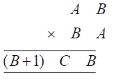Solution: Here, B×A= A i.e. A=1

Now first digit (B+1)

Where 1 can be carried from 1+B2 and becomes (B+1)(B2-9)B

∴ C= B2-1

Now B, B+1 and B2-9 are one single digit

This condition is satisfied for B=3 or B=4

For B<3, B2-9 is negative

For B >3, B2-9 will become two digit number

For B=3, C= 32– 9 = 9-9 = 0

For B=4, C= 42-9 = 16-9 = 7

∴ A=1, B= 3, C= 0

A=1, B=4, C=7

## Exercise 5D

Select the correct answer in each of the following

Q1. If 5×6 is exactly divisible by 3, then the least value of x is

a. 0
b. 1
c. 2
d. 3

Solution: we know that the sum of the digits of a number is divisible by 3 then the whole number is divisible by 3

5+x+6 = multiple of 3

11+x= 0, 3, 6, 9….

11+x= 12

∴ x=12-11 = 1

The least value of x is 1

Q2. If 64y8 is exactly divisible by 3, then the least value of y is

a. 0
b. 1
c. 2
d. 3

Solution: we know that the sum of the digits of a number is divisible by 3 then the whole number is divisible by 3

6+4+y+8 = multiple of 3

18+y= 0, 3, 6, 9….

18+y= 18

∴ y=18-18 = 0

The least value of y is 0

Q3. If 7×8 is exactly divisible by 9, then the least value of x is

a. 0
b. 2
c. 3
d. 5

Solution: we know that the sum of the digits of a number is divisible by 9 then the whole number is divisible by 9

7+x+8 = multiple of 9

15+x= 0, 9, 18, 27….

15+x= 18

∴ x=18-15 = 3

The least value of x is 3

Q4. If 37y4 is exactly divisible by 9, then the least value of y is

a. 2
b. 3
c. 1
d. 4

Solution: We know that the sum of the digits of a number is divisible by 9 then the whole number is divisible by 9

3+7+y+4 = multiple of 9

14+y= 0, 9, 18, 27….

14+y= 18

∴ y=18-14 = 4

The least value of y is 4

## RS Aggarwal Solutions for Class 8 Maths Chapter 5 – Playing with Numbers

Chapter 5- Playing with Numbers contains 4 exercises and the RS Aggarwal solutions present on this page provide the solutions for the questions present in each exercise. Now, let us have a look at the concepts discussed in this chapter.

• Numbers in Generalised form.
• Various Tests of Divisibility.
• Replacing alphabets with suitable numerals.

Exercise 5A

Exercise 5B

Exercise 5C

Exercise 5D

### Chapter Brief of RS Aggarwal Solutions for Class 8 Maths Chapter 5 – Playing with Numbers

RS Aggarwal Solutions for Class 8 Maths Chapter 5 – Playing with Numbers ensures that the students are thorough and familiar with the concepts. Regular revision of important concepts and formulas over time to time is the best way to strengthen your concepts. The solutions are designed in such a way that they are easy to understand and solve.

As the Chapter is about numbers, one must develop the ability to play with the numbers to be able to develop an interest and strengthen the foundation of the subject. In the RS Aggarwal Solutions book, many such exercise problems are given which enhances familiarity with the concepts.

#### 1 Comment

1. Deepanshu

It is very good